Parallel (Othographic & Oblique) Projection in Computer Graphics

• Difficulty Level : Easy
• Last Updated : 17 Mar, 2021

Projection is a kind of phenomena that are used in computer graphics to map the view of a 3D object onto the projecting display panel where the viewing volume is specified by the world coordinate and then map these world coordinate over the view port.

Projection is of the following kind :

a) Parallel Projection               b) Perspective Projection

Parallel Projection : Parallel projection is a kind of projection where the projecting lines emerge parallelly from the polygon surface and then incident parallelly on the plane. In parallel projection, the centre of the projection lies at infinity. In parallel projection, the view of the object obtained at the plane is less-realistic as there is no fore-shortcoming. and the relative dimension of the object remains preserves.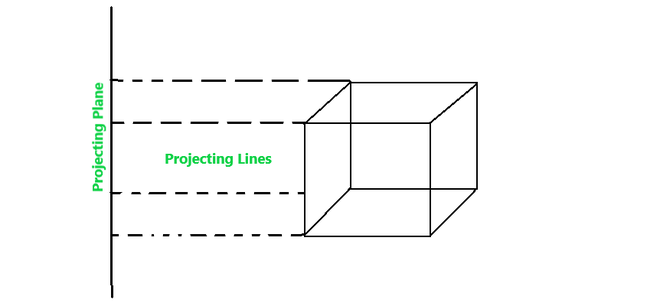Parallel projection is further divided into two categories :

a) Orthographic Projection

b) Oblique Projection

(a) Orthographic Projection : It is a kind of parallel projection where the projecting lines emerge parallelly from the object surface and incident perpendicularly at the projecting plane.

Orthographic Projection is of two categories :

(a).1. Multiview Projection : It is further divided into three categories –

(1) Top-View : In this projection, the rays that emerge from the top of the polygon surface are observed.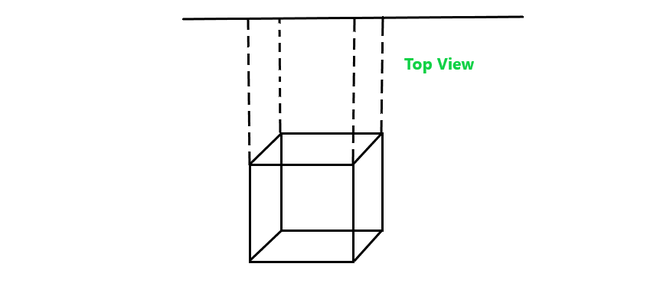2) Side-View : It is another type of projection orthographic projection where the side view of the polygon surface is observed.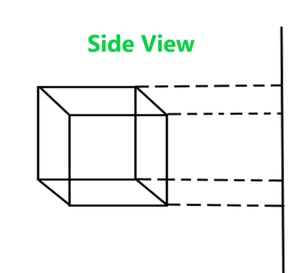3) Front-view : In this orthographic projection front face view of the object is observed.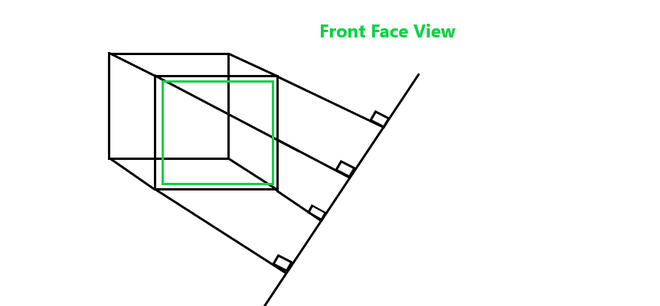a.2) Axonometric : Axonometric projection is an orthographic projection, where the projection lines are perpendicular to the plane of projection, and the object is rotated around one or more of its axes to show multiple sides.

It is further divided into three categories :

(1) Isometric Projection : It is a method for visually representing three-dimensional objects in two-dimensional display in technical and engineering drawings. Here in this projection, the three coordinate axes appear equally foreshortened and the angle between any two of them is 120 degrees.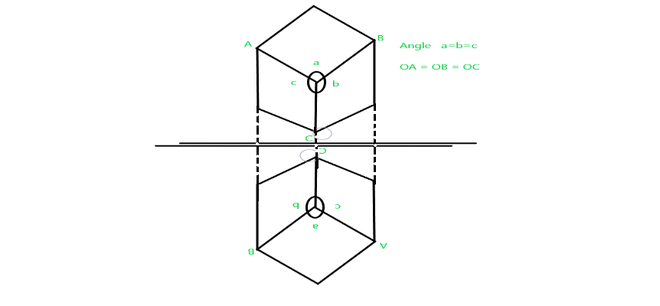(2) Dimetric Projection : It is a kind of orthographic projection where the visualized object appears to have only two adjacent sides and angles are equal.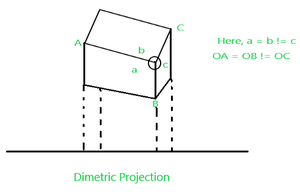(3) Trimetric Projection : It is a kind of orthographic projection where the visualized object appears to have all the adjacent sides and angles unequal.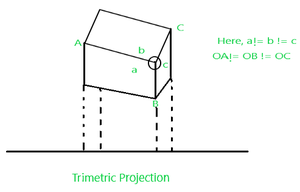(b) Oblique Projection : It is a kind of parallel projection where projecting rays emerges parallelly from the surface of the polygon and incident at an angle other than 90 degrees on the plane.

It is of two kinds :

(b).1. Cavalier Projection : It is a kind of oblique projection where the projecting lines emerge parallelly from the object surface and incident at 45‘ rather than 90′ at the projecting plane. In this projection, the length of the reading axis is larger than the cabinet projection.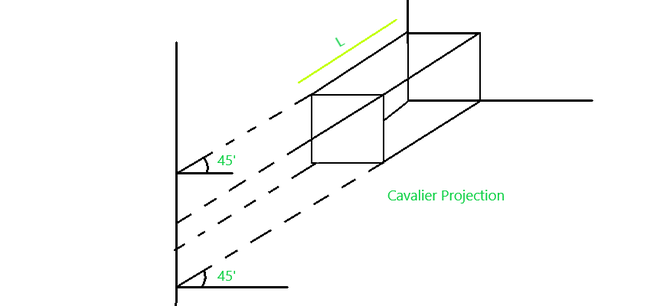(b). 2. Cabinet Projection : It is similar to that cavalier projection but here the length of reading axes just half than the cavalier projection and the incident angle at the projecting plane is 63.4′ rather 45′.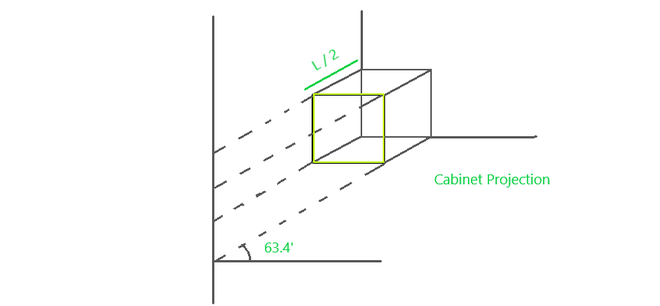My Personal Notes arrow_drop_up